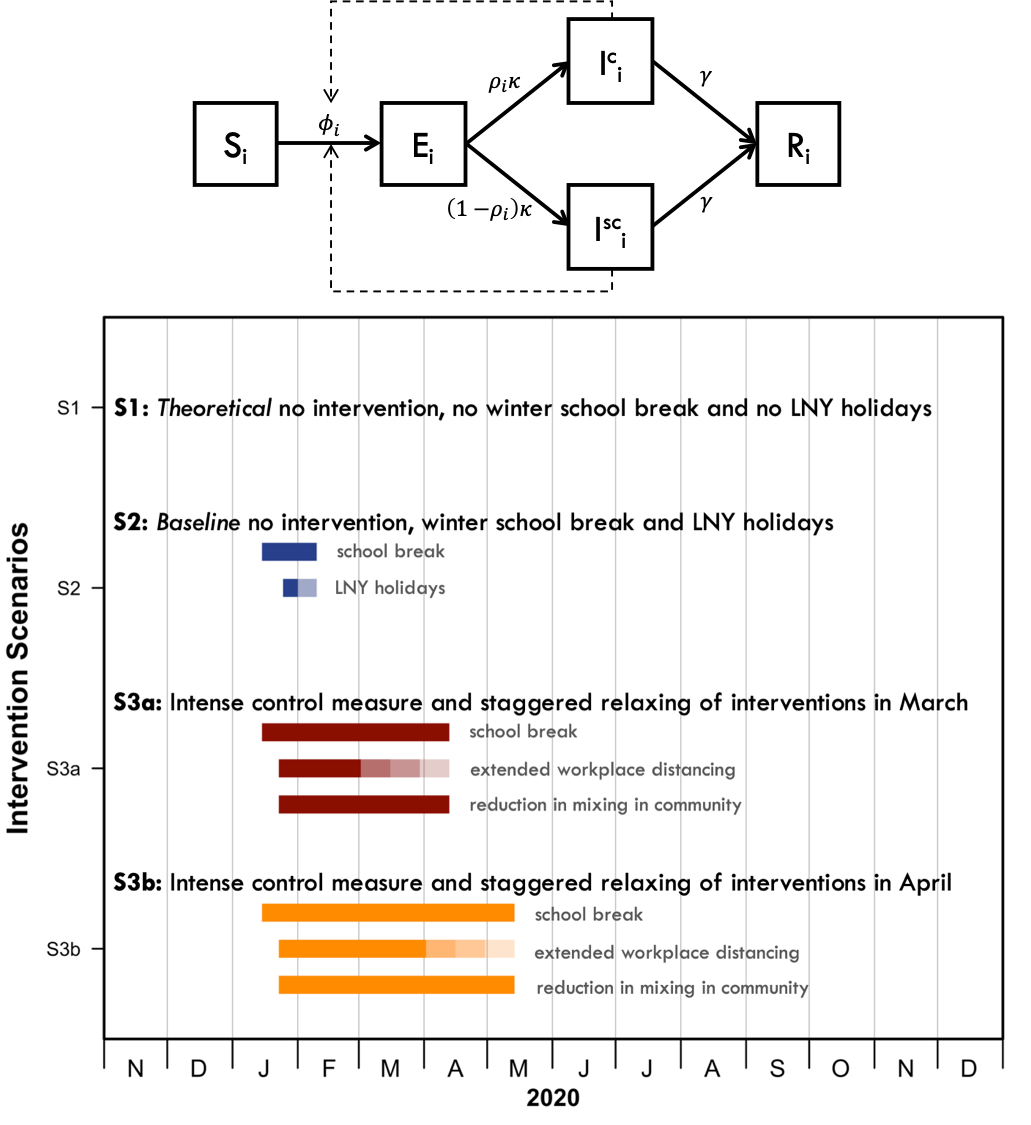# The effect of control strategies that reduce social mixing on outcomes of the COVID-19 epidemic in Wuhan, China: a modelling study

Status: Paper published at journal | First online: 18-03-2020 | Last update: 19-03-2020

The full paper accompanying this report can be found as a preprint here:

## Aim

To assess the impact of a range of control measures that reduce social mixing on data from the COVID-19 outbreak in Wuhan, China. Using the best estimates for the epidemiological parameters, we simulate the effects of the control measures using an age-structured, location-specific transmission model. The model uses data on contact patterns. Specifically, we adjust these patterns according to how they change under certain control measures. We then simulate our transmission model under these different scenarios and assess the impact.

## Introduction

The SARS-CoV-2, a novel coronavirus, emerged in the City of Wuhan, Hubei Province, China, in early December 2019.(1,2) Since then, the local and national governments have taken unprecedented measures in response to the COVID-19 outbreak caused by SARS-CoV-2.(3) Exit screening of passengers was shortly followed by travel restrictions in Wuhan on 23rd January 2020, halting all means of unauthorised travel into and out of the city. Similar control measures were extended to the entire province of Hubei by 26th January 2020.(3) Non-pharmaceutical social distancing interventions such as extended school closure and workplace distancing were introduced to reduce the impact of the COVID-19 outbreak in Wuhan.(4) Within the city, schools remained closed; Lunar New Year holidays were extended so that people stayed away from their workplaces; the local government promoted social distancing and encouraged residents to avoid crowded places. These measures are known to have greatly changed the age-specific mixing patterns within the population in previous outbreak response efforts for other respiratory infectious diseases.(5,6) While travel restrictions undoubtedly had a role in reducing the exportations of infections outside of Wuhan, and delayed the onset of outbreaks in other regions,(7,8) changes in the mixing patterns affected the trajectory of the outbreak within Wuhan itself. In order to estimate the effects of social distancing measures on the progression of the COVID-19 epidemic, we look at Wuhan, hoping to provide some insights for the rest of the world.

To examine how these changes in population mixing have affected the outbreak progression in Wuhan, we used synthetic location-specific contact patterns in Wuhan and adapted it in the presence of school closures, extended workplace closures, reduction in mixing in the general community. Using these matrices and the latest estimates of the epidemiological parameters of the Wuhan outbreak,(1,9,14–16) we simulated the ongoing trajectory of an outbreak in Wuhan using an age-structured susceptible-exposed-infected-removed (SEIR) model(17,18) for several social distancing measures.

## Methods

### SEIR Model

We simulated the outbreak in Wuhan using a deterministic stage-structured SEIR model over one year period, during which the modelled outbreak peters out. An implication of this approach is that all demographic changes in the population (i.e., births, deaths, and ageing) are ignored.

For a given age group , epidemic transitions can be described by:

$S_{i, t+1} = S_{i, t} - \beta S_{i, t} \sum_{j = 1}^n C_{i, j} I_{j, t}^c - \alpha \beta S_{i, t} \sum_{j = 1}^n C_{i, j} I_{j, t}^{SC}$

$E_{i, t+1} = \beta \sum_{j = 1}^n C_{i, j} I_{j, t}^C + \alpha \beta S_{i, t} \sum_{j = 1}^n C_{i, j} I_{j, t}^{SC} - (1 - \kappa) E_{i, t}$

$I_{i, t+1}^C = \rho_i \kappa E_{i, t} + (1 - \gamma)I_{j, t}^C$

$I_{j, t+1}^{SC} = (1 - \rho_i) \kappa E_{i, t} + (1 - \gamma)I_{j, t}^C$

$R_{i, t+1} = R_{i, t} + \gamma I_{j, t + 1}^C + \gamma I_{j, t + 1}^{SC}$

Where $$\beta$$ is the transmission rate (scaled to the right value of $$R_0$$), $$C_{i,j}$$ describe the contacts of age group $$j$$ made by age group $$i$$, $$\kappa = 1 - \exp(\frac{1}{d_L})$$ is the daily probability of exposed individual becoming infectious (with $$d_t$$ being the average incubation period), and $$\gamma = 1 - \exp(\frac{1}{d_I})$$ is the daily probability that an infected individual recovers when the average duration of infection is $$D_I$$. We further incorporated contributions of asymptomatic and subclinical cases, $$1 - \rho$$ denotes the probability of an infected cases being asymptomatic or subclinical. We assumed that younger individuals are more likely to be asymptomatic (or subclinical) and less infectious (proportion of infectiousness compared to $$I^C, \alpha$$ ).

Parameter Values References
Basic reproduction number, $$R_o$$ 2·2 (1·6–3·0) 1 Kucharski and colleagues 1, 4
Average incubation period, $$d_L$$ 6·4 days Backer and colleagues 1,5
Average duration of infection, $$d_I$$ 3 or 7 days Woelfel and colleagues22
Initial number of infected, $$I_O$$ 200 or 2000 Abbott and colleagues 16
Pr(infected case is clinical), $$\rho_i$$ 0 or 0·4, for Bi and colleagues20
0 or 0·8 for Davies and colleagues21
Pr(infection acquired from subclinical), $$\alpha$$ 0·25 Liu and colleagues 19Figure 1. Age-structured SEIR model and details of the modelled social distancing interventions. The age-specific mixing patterns of individuals in age group alter their likelihood of being exposed to the virus given a certain number of infectives in the population. Younger individuals are more likely to be asymptomatic and less infectious, i.e. subclinical (SC). When $$\rho_i = 0$$ for all $$i$$, the model simplifies to a standard SEIR. The force of infection $$\phi_{i, t}$$ is given by $$1 - (\beta \sum_j C_{i,j} I_{j,t}^C + \alpha \beta \sum_j C_{i,j} I_{j, t}^{SC})$$

## Results

Our simulations show that the control measures aimed at reducing the social mixing in the population can be effective in reducing the magnitude and delaying the peak of the outbreak. Figure 3 shows the effects of different control measures among individuals aged 55–60 and 10–15 years old. The standard school winter break and holidays for the Lunar New Year would have had little effect on progression of the outbreak had the school and workplace re-opened as normal.